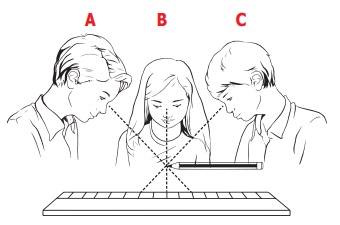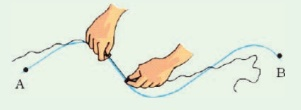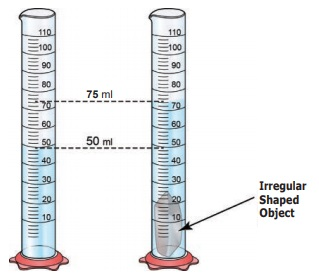Home | | Science 6th Std | Questions Answers

## Chapter: 6th Science : Term 1 Unit 1 : Measurements

6th Science : Term 1 Unit 1 : Measurements : Book Back Questions Answers, Solution

Evaluation

1. The girth of a tree can be measured by

a. Metre scale

b. Metre rod

c. plastic ruler

d. measuring tape

2. The conversion of 7 m into cm gives

a. 70 cm

b. 7 cm

c. 700 cm

d. 7000 cm

3. Quantity that can be measured is called

a. Physical quantity

b. Measurement

c. unit

d. motion

4. Choose the correct one

a. km > mm > cm > m

b. km> mm > cm > km

c. km > m > cm > mm

d. km > cm > m > mm

5. While measuring length using a ruler, the position of your eye should be

a. Left side of the point.

b. Vertically above the point where the measurement is to be taken.

c. Right side of the point

d. Any where according to one’s convenience.

II. True or False

1. 126 kg is the correct way of expressing mass. [True]

2. Length of one’s chest can be measured by using metre scale. [True]

3. Ten millimetres makes one centimetre. [True]

4. A hand span is a reliable measure of length. [False]

5. The SI system of units is accepted everywhere in the world. [True]

III. Fill up the blanks

1. SI Unit of length is symbolically represented as meter(m).

2. 500 gm = 1/2 kilogram

3. Distance between Delhi and Chennai can be measured in Kilometer

4. 1 m = 100 cm

5. 5 km = 5000 m.

IV. Analogy

1. Sugar: Beam balance; Lime juice?  [ Answer: beaker ]

3. Milk: volume; vegetables? [ Answer: mass ]

V. Match the following

Column A / Column B

1. Length of the fore arm - Metre

2. SI unit of length- Second

3. Nano- 103

4. SI Unit of time - 10–9

5. Kilo- Cubit

Column A - Column B

1. Length of the fore arm - Cubit

2. SI unit of length - Metre

3. Nano - 10-9

4. SI Unit of time - Second

5. Kilo - 103

VI. Complete the given table

Volume = litre : Kg = mass

Length of your little finger = hand span :  Km : length.

VII. Arrange in increasing order of unit

1 Metre,  1 centimetre, 1 kilometre, and 1 millimetre.

Answer: 1 millimetre , 1 centimetre , 1 Metre , 1 kilometre.

VIII. Find the answer for the ­following questions within the grid

1. 10-3 is one milli.

2. SI Unit of time second.

4. Time is the one what a clock reads

5. Mass is the amount of substance present in an object

6. Average can be taken to get the final reading of the recordings of different of students for a single measurement.

7. Length is a fundamental quantity

8. Odometer shows the distance covered by an automobile

9. A tailor use tape to take measurements to stitch a cloth

10. Liquids are measured with this physical quantity litreIX. Answer in a word or two.

1. What is the full form of SI system?

The full form of SI system is International Standard

2. Name any one instrument used for measuring mass.

Instrument used for measuring mass is electronic balance

3. Find the odd one out Kilogram, Millimetre, Centimetre, Nanometre

Kilogram, Millimetre, Centimetre, Nanometre

Kilogram

4. What is the SI Unit of mass?

The SI Unit of mass is Kilogram.

5. What are the two parts present in a measurement

The two parts present in a measurement are a number and its unit.

X. Answer in a sentence or two.

1. Define measurement.

The comparison of unknown quantities with some known quantities is known as measurement.

Measurement of a quantity has two parts: a number and its unit.

E.g., 500 gm , 5 km

2. Define mass.

Mass is the measure of the amount of matter in an object.

Weight is the gravitational pull experienced by the mass.

3. The distance between two places is 43.65 km. Convert it into metre and cm.

1 km = 1000 m

43.65 km = 43.65 × 1000 = 43650 m

1 m = 100 cm ; 1 km = 100000 cm

43.65 km = 43.65 × 100000 = 4365000 cm

4. How will you measure the volume of irregular objects?

There is no mathematical formula to measure the volume of irregular objects.

By water displacement method we measure the volume of irregular objects.

5. What are the rules to be followed to make accurate measurement with scale?

Correct position of the eye is important for taking measurement.

Your eye must be exactly in front of vertically above the point where the measurement has to be taken.In the above representation, to avoid parallax error, reading will be correct.

From positions ‘A’ and ‘C’, the readings will be different and erroneous.

B is Correct position of the eye to take measurement.

XI. Solve the following

1. The distance between your school and your house is 2250 m. Express this distance in kilometre.

Solution: 1 km = 1000 m

2250 m = 2250 / 1000 = 2.25 km

2. While measuring the length of a sharpened pencil, reading of the scale at one end is 2.0 cm and at the other end is 12.1 cm. What is the length of the pencil?

Given: one end (starting) = 2.0 cm

other end (finishing) = 12.1 cm

To find: the length of the pencil = ?

Solution: The length of the pencil = finishing end - starting end

= 12.1 - 2.0 cm = 10.1 cm

The length of the pencil is 10.1cm.

XII. Write in detail

1. Explain two methods those you can use to measure the length of a curved line.

Measuring the length of a curved line Using a string

Draw a curved line AB on a piece of paper.Place a String along the curved line.

Make sure that the string covers every bit of the curved line.

Mark the points where the curved line begins and ends on the string.

Now, stretch the string along the length of a meter scale and measure the distance between the two markings of the string.

Note it. This will give you the length of a curved line.

2. Measuring the length of a curved line using a divider.

Draw a curved line AB on a piece of paper

Separate the legs of the divider by 0.5 cm or 1 cm using a ruler.Place it on the curved line starting from one end. Mark the position of the other end.

Move it along the line again and again cutting the line into number, of segments of equal lengths. The remaining-parts of the line can be measured using a scale.

Count the number of segments

Therefore, the length of the line = (number of segments × length of each segment) + length of the left over part.

3. How will you measure the volume of irregular objects?

There is no mathematical formula to measure the volume of irregular objects.

But there are many ways to find volume of irregular objects.

Water displacement method:

Fill a graduated measuring cylinder with water to certain level, say 50 ml.

Tie the stone with a piece of fine thread. Immerse the stone completely into water.

As the stone is immersed, we can observe that water level increases.

The stone displaces the water to occupy the space inside the measuring cylinder.

It would be equal to the space taken up by stone.

So the amount of water displaced will be the volume of the stone.

This method is called as water displacement method.Suppose initially the water level was 50 ml.

After you immerse a stone the water level rises to 75 ml.

Volume of the water displaced is 75 ml - 50 ml = 25ml

1 ml = 1 cubic cm

25ml = 25 cm.the volume of stone = 25cm3.

4. Fill up the following chart.Tags : Measurements | Term 1 Unit 1 | 6th Science , 6th Science : Term 1 Unit 1 : Measurements
Study Material, Lecturing Notes, Assignment, Reference, Wiki description explanation, brief detail
6th Science : Term 1 Unit 1 : Measurements : Questions Answers | Measurements | Term 1 Unit 1 | 6th Science